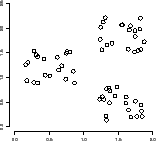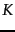Next: Clustering in information retrieval Up: irbook Previous: References and further reading   Contents   Index

# Flat clustering

Clustering algorithms group a set of documents into subsets or clusters . The algorithms' goal is to create clusters that are coherent internally, but clearly different from each other. In other words, documents within a cluster should be as similar as possible; and documents in one cluster should be as dissimilar as possible from documents in other clusters.Clustering is the most common form of unsupervised learning . No supervision means that there is no human expert who has assigned documents to classes. In clustering, it is the distribution and makeup of the data that will determine cluster membership. A simple example is Figure 16.1 . It is visually clear that there are three distinct clusters of points. This chapter and Chapter 17 introduce algorithms that find such clusters in an unsupervised fashion.

The difference between clustering and classification may not seem great at first. After all, in both cases we have a partition of a set of documents into groups. But as we will see the two problems are fundamentally different. Classification is a form of supervised learning (Chapter 13 , page 13.1 ): our goal is to replicate a categorical distinction that a human supervisor imposes on the data. In unsupervised learning, of which clustering is the most important example, we have no such teacher to guide us.

The key input to a clustering algorithm is the distance measure. In Figure 16.1 , the distance measure is distance in the 2D plane. This measure suggests three different clusters in the figure. In document clustering, the distance measure is often also Euclidean distance. Different distance measures give rise to different clusterings. Thus, the distance measure is an important means by which we can influence the outcome of clustering.

Flat clustering creates a flat set of clusters without any explicit structure that would relate clusters to each other. Hierarchical clustering creates a hierarchy of clusters and will be covered in Chapter 17 . Chapter 17 also addresses the difficult problem of labeling clusters automatically.

A second important distinction can be made between hard and soft clustering algorithms. Hard clustering computes a hard assignment - each document is a member of exactly one cluster. The assignment of soft clustering algorithms is soft - a document's assignment is a distribution over all clusters. In a soft assignment, a document has fractional membership in several clusters. Latent semantic indexing, a form of dimensionality reduction, is a soft clustering algorithm (Chapter 18 , page 18.4 ).

This chapter motivates the use of clustering in information retrieval by introducing a number of applications (Section 16.1 ), defines the problem we are trying to solve in clustering (Section 16.2 ) and discusses measures for evaluating cluster quality (Section 16.3 ). It then describes two flat clustering algorithms,-means (Section 16.4 ), a hard clustering algorithm, and the Expectation-Maximization (or EM) algorithm (Section 16.5 ), a soft clustering algorithm.-means is perhaps the most widely used flat clustering algorithm due to its simplicity and efficiency. The EM algorithm is a generalization of-means and can be applied to a large variety of document representations and distributions.

SubsectionsNext: Clustering in information retrieval Up: irbook Previous: References and further reading   Contents   Index